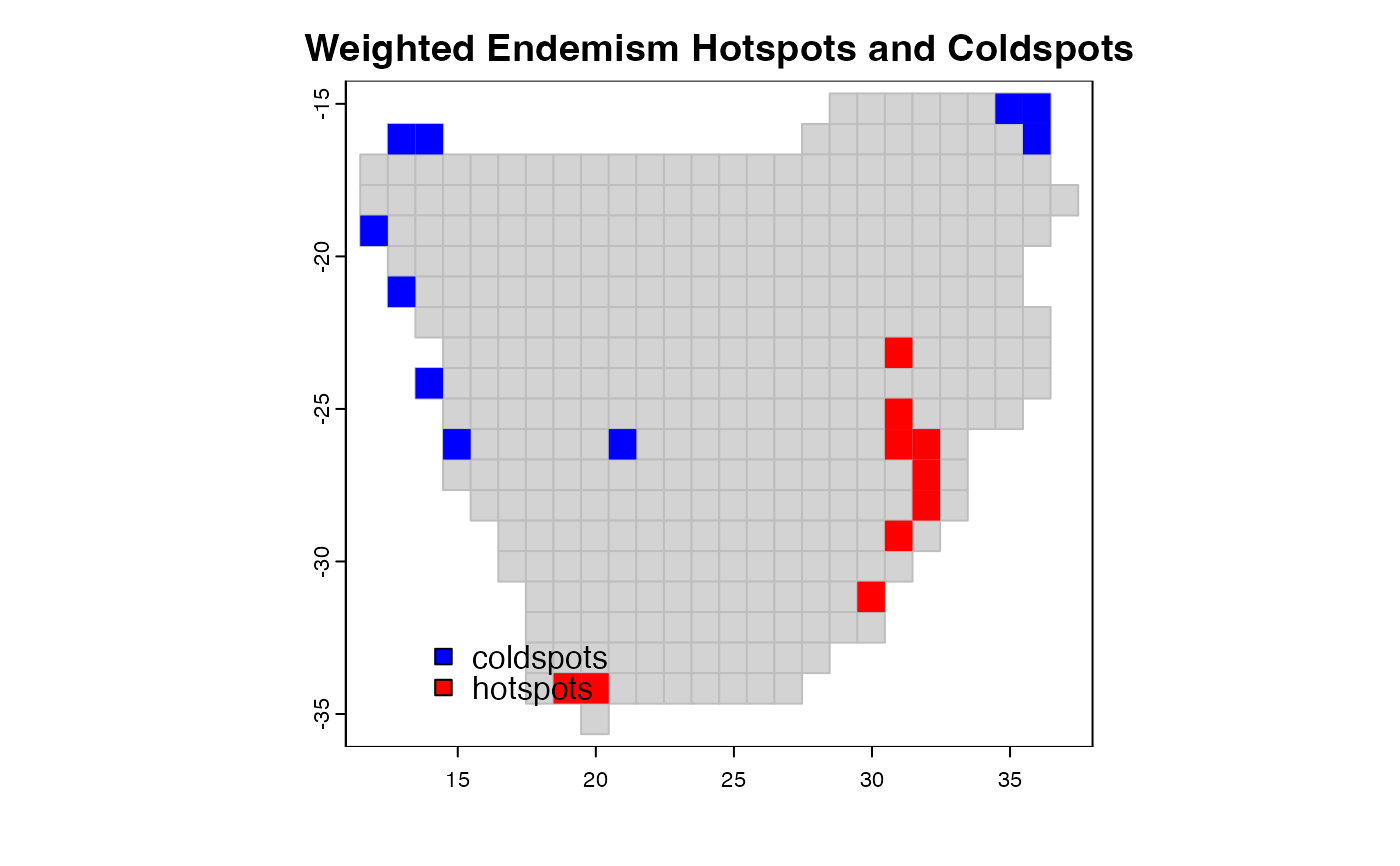coldspots and hotspots map areas or grid cells with lowest or highest values, respectively, of a biodiversity metric e.g. species richness, species endemism or degree of threat.

coldspots(x, prob = 2.5, na.rm = TRUE, ...)

hotspots(x, prob = 2.5, na.rm = TRUE, ...)

## Arguments

x

a vector on which to compute coldspots

prob

The threshold quantile for representing the lowest (coldspots) or highest (hotspots) proportion of biodiversity in an area. By default, the threshold is set to prob = 2.5 percent.

na.rm

logical; if true, any NA and NaN's are removed from x before the quantiles are computed.

...

Further arguments passed to or from other methods.

## Value

A vector of integers of 1s and 0s with 1 corresponding to the coldspots or hotspots

## Author

Barnabas H. Daru darunabas@gmail.com

## Examples

library(terra)
data(africa)
p <- vect(system.file("ex/sa.json", package = "phyloregion"))

Endm <- weighted_endemism(africa$comm) C <- coldspots(Endm) # coldspots H <- hotspots(Endm) # hotspots ## Merge endemism values to shapefile of grid cells. DF <- data.frame(grids=names(C), cold=C, hot=H) m <- merge(p, DF, by = "grids", all = TRUE) plot(p, border = "grey", col = "lightgrey", main = "Weighted Endemism Hotspots and Coldspots") plot(m[(m$cold == 1), ], col = "blue", add = TRUE, border = NA)
plot(m[(m\$hot == 1), ], col = "red", add = TRUE, border = NA)
legend("bottomleft", fill = c("blue", "red", "yellow", "green"),
legend = c("coldspots", "hotspots"), bty = "n", inset = .092)